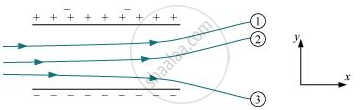# The Figure Shows Tracks of Three Charged Particles in a Uniform Electrostatic Field. Give the Signs of the Three Charges. Which Particle Has the Highest Charge to Mass Ratio? - Physics

The figure shows tracks of three charged particles in a uniform electrostatic field. Give the signs of the three charges. Which particle has the highest charge to mass ratio?#### Solution

Opposite charges attract each other and the same charges repel each other. It can be observed that particles 1 and 2 both move towards the positively charged plate and repel away from the negatively charged plate. Hence, these two particles are negatively charged. It can also be observed that particle 3 moves towards the negatively charged plate and repels away from the positively charged plate. Hence, particle 3 is positively charged.

The charge to mass ratio (emf) is directly proportional to the displacement or amount of deflection for a given velocity. Since the deflection of particle 3 is the maximum, it has the highest charge to mass ratio.

Concept: Electric Field - Electric Field Due to a System of Charges
Is there an error in this question or solution?
Chapter 1: Electric Charges and Fields - Exercise [Page 47]

#### APPEARS IN

NCERT Physics Part 1 and 2 Class 12
Chapter 1 Electric Charges and Fields
Exercise | Q 1.14 | Page 47
NCERT Class 12 Physics Textbook
Chapter 1 Electric Charge and Fields
Exercise | Q 14 | Page 47
Share